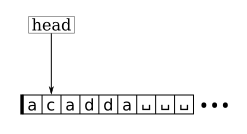Math and science::Theory of Computation

# Turing machine

### Conceptual description: finite state machine + tape

A Turing machine can be thought of as a finite state machine that controls a reading/writing head that moves along a a tape.#### The tape, head and state machine

The tape is a long physical 1-dimensional storage device. It has a head which is an object positioned at one of the storage cells; the head is capable of reading and writing to a cell and capable of moving 1 position forward or back inbetween read/write operations. The tape is infinite in one direction. Typically, the tape is considered to start at a zero position and extend to the right indefinitely. The head moves along the tape reading charcaters from it and writing characters to it. The head's movement (including whether to stop moving) is determined by a state machine.

Reading produces a character from the tape at the head position. This character becomes the next input to the finite state machine. Writing places a character on the tape at the position of the head. The character written is chosen by the finite state machine. The character replaces the existing sequence element. Note that the finite machine is provided the character on the tape as input but it doesn't get the tape head's location as input.

In addition to writing a character to the tape, the finite state machine's also outputs an element from a binary set which encodes whether the head should move left or right once it has finished writing at the current tape location. Usually this the set is described as containing the two characters 'L' and 'R'; L decrements the tape head position, R increments it. When the head is at the beginning of the tape, L has no effect.

### Formal definition

The formal definition of a Turing machine builds off of the definition of a finite automata. The definition of finite automata is recalled here.

#### Finite automata

A finite automaton is a 5-tuple ($$Q, \Sigma, q_0, F, \delta$$), where:

1. $$Q$$ is a finite set called the states,
2. $$\Sigma$$ is a finite set called the alphabet,
3. $$q_0 \in Q$$ is the start sate, and
4. $$F \subseteq Q$$ is the set of accept states.
5. $$\delta : Q \times \Sigma \to Q$$ is the transition function.

The definition of the Turing machine modifies the above definition of a finite automaton in three ways:

Specify a blank symbol
A symbol is introduces that is only present on the tape (it is not a symbol that can be present in the input). A Turing machine uses this symbol to, among other things, detect the end of the input once the input is placed on the tape.
Change to the transition function
Secondly, it modifies the transision function by increasing the dimensionality of its codomain. The codomain becomes [$$Q \times ? \times ?$$ ].
Introduction of a reject state
A reject state is similar to an accept state. The addition of a second type of ending state allows for the machine to end while indicating a sense of failure.

With these changes, the definition of a Turing machine looks like:

### Turing machine

A Turing machine is parameterized as a 7-tuple, $$(Q, \Sigma, \Gamma, \delta, q_{accept}, q_{reject})$$, where $$Q, \Sigma$$ and $$\Gamma$$ are all finite sets, $$\delta$$ is a function and $$q_{accept}$$ and $$q_{reject}$$ are elements of $$\Sigma$$.

1. $$Q$$ is the set of states of the machine's underlying finite state machine.
2. $$\Sigma$$ is the input alphabet. It doesn't contain the [...].
3. $$\Gamma = \Sigma \cup \{␣\}$$ is [what alphabet?].
4. [$$\delta : Q \times ? \to ? \times ? \times ?$$] is the transition function.
5. $$q_0 \in Q$$ is the start state.
6. $$q_{accept} \in Q$$ is the accept state.
7. $$q_{reject} \in Q$$ is the reject state, where $$q_{reject} \ne q_{accept}$$.

There is a number of ways to denote a Turing machine with a tuple of sets. All are functionally equivalent but differ in how much of the machines specification is included in the tuple. The above formulation closely follows the presentation of Michael Sipser. The reverse side contains a discussion of some of the issues with this and other definitions.